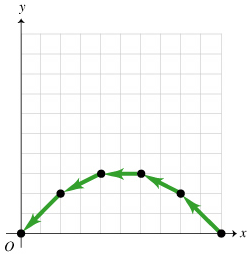# Problem: For the motion diagram given (Figure 1), sketch the shape of a possible graph for the x velocity versus time, vx(t). Use the indicated coordinate system. One unit of time elapses between consecutive dots in the motion diagram.

###### FREE Expert Solution

The change in x from one dot to the other is constant implying a constant horizontal velocity.

x is moving with a constant negative velocity.

91% (401 ratings)###### Problem Details

For the motion diagram given (Figure 1), sketch the shape of a possible graph for the x velocity versus time, vx(t). Use the indicated coordinate system. One unit of time elapses between consecutive dots in the motion diagram.